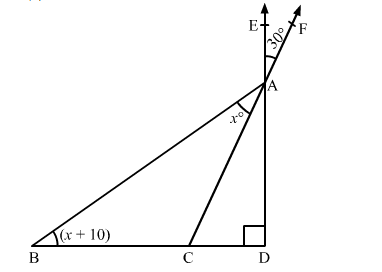# The the given figure, EAD ⊥ BCD. Ray FAC cuts ray EAD at a point A such that ∠EAF = 30°. Also,

Question:

The the given figure, EAD  BCD. Ray FAC cuts ray EAD at a point A such that ∠EAF = 30°. Also, in ∆BAC, BAC x° and ∠ABC = (x + 10)°. Then, the value of x is
(a) 20
(b) 25
(c) 30
(d) 35Solution:

In the given figure, ∠CAD = ∠EAF            (Vertically opposite angles)

In ∆ABD,

⇒ (x + 10)° + (x° + 30°) + 90° = 180°

⇒ 2x° + 130° = 180°

⇒ 2x° = 180° − 130° = 50°

⇒ x = 25

Thus, the value of x is 25.

Hence, the correct answer is option (b).